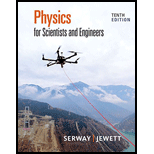# A certain lightbulb is rated at 60.0 W when operating at an rms voltage of 120 V. (a) What is the peak voltage applied across the bulb? (b) What is the resistance of the bulb? (c) Does a 100-W bulb have greater or less resistance than a 60.0-W bulb? Explain.### Physics for Scientists and Enginee...

10th Edition
Raymond A. Serway + 1 other
Publisher: Cengage Learning
ISBN: 9781337553278

#### Solutions

Chapter
Section### Physics for Scientists and Enginee...

10th Edition
Raymond A. Serway + 1 other
Publisher: Cengage Learning
ISBN: 9781337553278
Chapter 32, Problem 2P
Textbook Problem
24 views

## A certain lightbulb is rated at 60.0 W when operating at an rms voltage of 120 V. (a) What is the peak voltage applied across the bulb? (b) What is the resistance of the bulb? (c) Does a 100-W bulb have greater or less resistance than a 60.0-W bulb? Explain.

(a)

To determine
The peak voltage applied across the bulb.

### Explanation of Solution

Given Information: Average power of the light bulb is 60.0W , root mean square voltage across the power source is 120V .

Formula to calculate the peak voltage applied across the bulb is,

Vpeak=2Vrms (1)

Here,

Vpeak is the peak voltage applied across the bulb.

Vrms is the root mean square voltage across the power source

(b)

To determine
The resistance of the light bulb.

(c)

To determine
Whether a 100W light bulb have greater or less resistance than a 60.0W light bulb.

### Still sussing out bartleby?

Check out a sample textbook solution.

See a sample solution

#### The Solution to Your Study Problems

Bartleby provides explanations to thousands of textbook problems written by our experts, many with advanced degrees!

Get Started

Find more solutions based on key concepts
In what other areas of the world are fjords common?

Fundamentals of Physical Geography

A deficiency of niacin may result in which disease? pellagra beriberi scurvy rickets

Nutrition: Concepts and Controversies - Standalone book (MindTap Course List)

Why cant you see deeper into the Sun than the photosphere?

Foundations of Astronomy (MindTap Course List)

Outline the main points in the condensation theory of star and planet formation.

Oceanography: An Invitation To Marine Science, Loose-leaf Versin